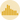# Basic histogram in Matplotlib

This guide explains how to create a simple histogram with Matplotlib. Several examples are included to show how it is possible to modify a variety of details.

## Most basic histogram

First of all, let's import Matplotlib and Numpy, two widely used libraries for data visualization and data wrangling.

``````import matplotlib.pyplot as plt
import numpy as np # only used to compute a median value``````

Now, let's pretend the following are weekly hours of work reported by people in a survey. This is the dataset required to build a histogram: an array of numeric value. Note that it could also be a column of a `pandas` data frame.

``hours = [17, 20, 22, 25, 26, 27, 30, 31, 32, 38, 40, 40, 45, 55]``

Creating a histogram is as simple as calling `plt.hist(hours)` or using `ax.hist(hours)` with Matplotlib's object-oriented interface:

``````# Initialize layout
fig, ax = plt.subplots(figsize = (9, 9))

# Make histogram
ax.hist(hours);``````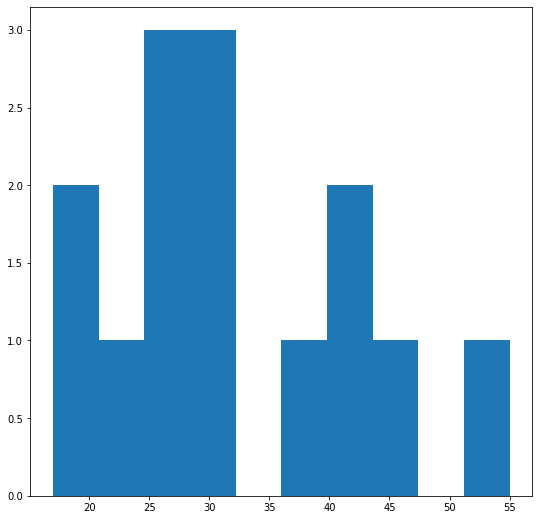## Specify the number of bins

One problem is that we are not certain about the binning being used. Fortunately, it is possible to specify the binning by passing an integer that specifies the number of bins, or a list of values that represent the bins.

``````fig, ax = plt.subplots(figsize = (9, 6))
# Use 5 bins
ax.hist(hours, bins=5);``````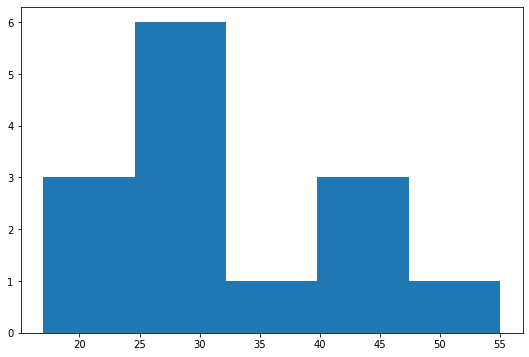## Color edges

The chart may not be clear because there's nothing separating the bins. Let's specify a color for the edges with the `edgecolor` argument.

``````fig, ax = plt.subplots(figsize = (9, 6))
ax.hist(hours, bins=5, edgecolor="black");``````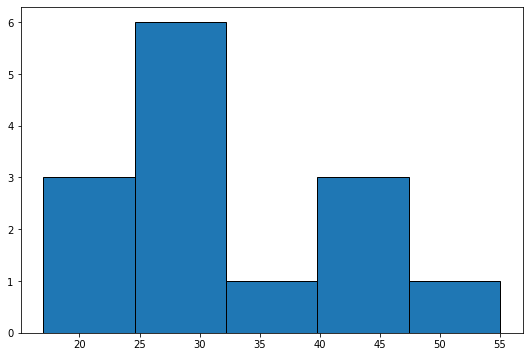Now the bins are much clearer. Let's see how it looks when we pass a list of values for the bins:

``````bins = [10, 20, 30, 40, 50, 60]
fig, ax = plt.subplots(figsize = (9, 6))
ax.hist(hours, bins=bins, edgecolor="black");``````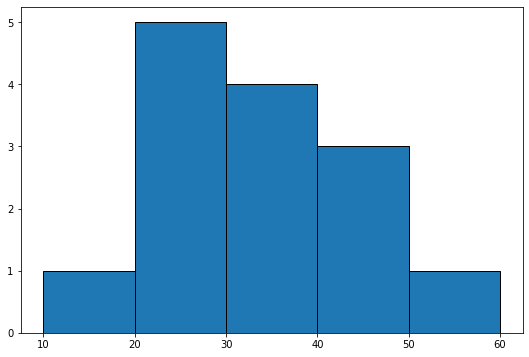## Zoom on a specific sample

It's possible to remove a particular bin. That will also remove the values from the data that fall in that bin. Values smaller than 20 won't be included in the following histogram.

``````bins = [20, 30, 40, 50, 60]
fig, ax = plt.subplots(figsize = (9, 6))
ax.hist(hours, bins=bins, edgecolor="black");``````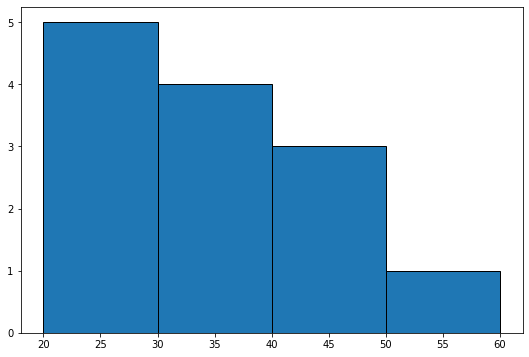And finally, let's see how to add a vertical line indicating some interesting quantity. In this case, the line is going to reprsent the median hours of work per week.

Note: read this specific blogpost of the gallery for more on matplotlib annotation.

``````median_hour = np.median(hours)
bins = [10, 20, 30, 40, 50, 60]

fig, ax = plt.subplots(figsize = (6, 6))
ax.hist(hours, bins=bins, edgecolor="black", color="#69b3a2", alpha=0.3)

# axvline: axis vertical line
ax.axvline(median_hour, color="black", ls="--", label="Median hour")
ax.legend();``````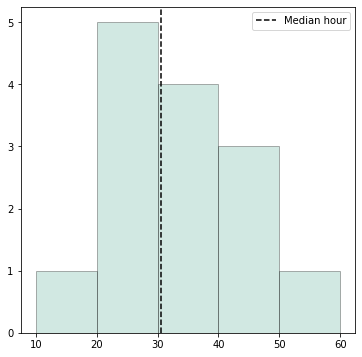## Contact & Edit

👋 This document is a work by Yan Holtz. Any feedback is highly encouraged. You can fill an issue on Github, drop me a message onTwitter, or send an email pasting `yan.holtz.data` with `gmail.com`.